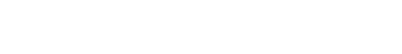# Hello, students### APM466/MAT1856 first blog

Firstly, in the equation of dirty price, is r the discount rate?

Not really; note that when we look at a bond price, we only see market prices: r is the yield of the bond

I was also wondering if the dirty price is the price of cash flow from last payment date to the maturity,

Dirty price is the present values of future cashflows TODAY

accrued interest is the price of interest from last payment date to the present

No: accrued interest is the corresponding share of the future coupon payments to the buyer

and clean price is the price of cash flow from the present to the maturity?

No; clean price is the transaction price (excluding accrued interest)

And why do we use the instantaneous compounding to calculate the rate of zero coupon bonds? Why do we use zero coupon rates and instantaneous compounding to get the price of future cash flow? Is that because of no arbitrage?

No, we do that for convenience, we could have taken any other compounding criteria, the formulas would then depend on the compounding